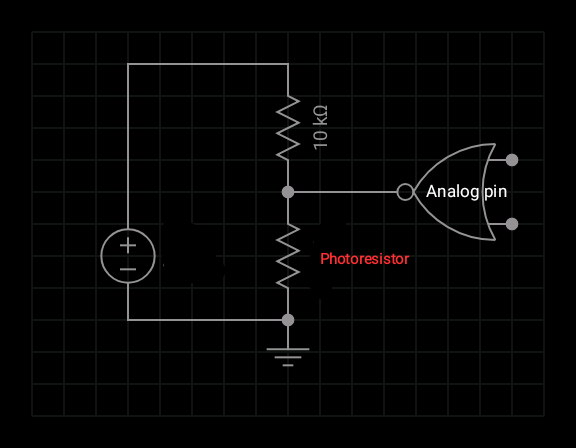# Sun tracker on blynk

Hi,Blynkers!
Today I want to tell you about the processing of data obtained from the photoresistor.The resistance of the photoresistor has a logarithmic dependence on brightness. Let me remind you that brightness is the amount of light falling on the area. You should have learned about the logarithm in school. Separately, it should be noted that the resistance of the photoresistor will be different at the same brightness, but in different colors, that is, the wavelengths of light. Let’s see how to connect it to Arduino.And now the math and the code:

``````int lux(int d)
{
float R = (float)((d * 10000) / (1024 - d));
int Luxi = int(round(pow(10, (log10(r1 / R) / 0.67))));
return Luxi ;
}

``````

This function takes the value of an analog pin and returns the brightness in lux.To use it, insert it outside the functions loop and setup. And declare a macro at the beginning of the code.

``````#define r1 200000
``````

Where 200000 is the resistance of the photoresistor in the dark in ohms.
I use this function like this:

``````Blynk.virtualWrite(V5, lux( analogRead(A1)));
``````

I sent the timer values ​​of brightness to the server and this is what happened.

It was a cloudy evening. Brightness in dozens of lux, because in superchart you cannot manually set a redistribution over 9999.

1 Like

This is what one of my weeks looked like.

I also noticed an interesting effect after airing the room. This is similar to how a capacitor is charged and discharged, but it is just moisture (blue) and temperature (yellow).